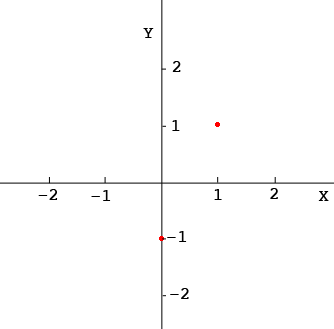SEARCH HOMEMath Central Quandaries & QueriesQuestion from Himanshu: Hi I Himanshu I having 1 doubt on 1 question this question of graph how to draw y=2x-1Hi,

I would use some graph paper and plot a few points. For example if $x = 0$ then $y = 2 \times 0 - 1 = -1$ and hence $(0, -1)$ is on the graph. Now try $x = 1.$ When $x = 1, y = 2 \times 1 - 1 = 1$ and hence $(1, 1)$ is on the graph.Plot a few more points. For example $x = 2, x = -1$ and a few more. What do you think is the shape of the graph? Can you draw it?

Penny* Registered trade mark of Imperial Oil Limited. Used under license.Math Central is supported by the University of Regina and the Imperial Oil Foundation.